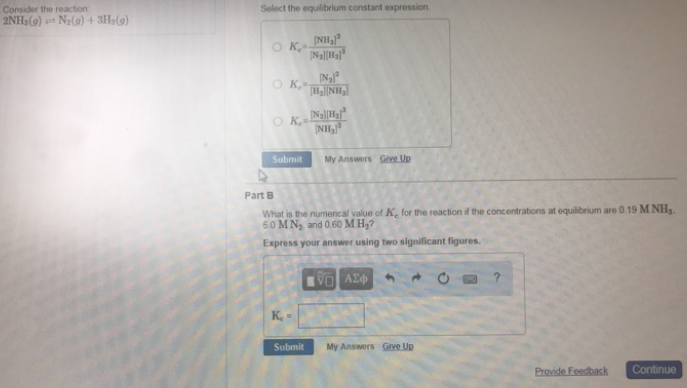Chemistry Practice Problems Equilibrium Expressions Practice Problems Solution: Consider the reaction: 2NH3 (g) ⇌ N2 (g) + 3H2 (g)...

🤓 Based on our data, we think this question is relevant for Professor Van Dorn's class at ARIZONA.

# Solution: Consider the reaction: 2NH3 (g) ⇌ N2 (g) + 3H2 (g) Select the equilibrium constant expression. (A) Kc = [NH3]2/[N2][H2]3 (B) Kc = [N2]2/[H2][NH3] (C) Kc = [N2][H2]3/[NH3]2 What is the numerical value of Kc for the reaction if the concentrations at equilibrium are 0.19 M NH3, 5.0 M N2, and 0.60 M H2? Express your answer using two significant figures.

###### Problem

Consider the reaction:

2NH3 (g) ⇌ N2 (g) + 3H2 (g)

Select the equilibrium constant expression.

(A) Kc = [NH3]2/[N2][H2]3

(B) Kc = [N2]2/[H2][NH3

(C) Kc = [N2][H2]3/[NH3]2

What is the numerical value of Kc for the reaction if the concentrations at equilibrium are 0.19 M NH3, 5.0 M N2, and 0.60 M H2View Complete Written Solution

Equilibrium Expressions

Equilibrium Expressions

#### Q. Consider the following reaction. A (aq) ⇌ 3B (aq)      Kc = 2.78 x 10-6 at 500 K If a 4.50 M sample of A is heated to 500 K, what is the concentration...

Solved • Tue Jul 10 2018 13:08:46 GMT-0400 (EDT)

Equilibrium Expressions

#### Q. For the reaction N2 (g) + 3H2 (g) ⇌ 2NH3 (g) what is the value of Kc at 500°C if the equilibrium concentrations are as follows: [H 2] = 0.30 M, [N2] =...

Solved • Mon Jul 09 2018 14:19:54 GMT-0400 (EDT)

Equilibrium Expressions

#### Q. What is the Kc for the following equilibrium at 1000°C, CO (g) + 3H2 (g) ⇌ CH4 (g) + H2O (g) [H2] = 0.25 M, [CO] = 0.60 M, [CH 4] = 1.9 M, and [H2O] =...

Solved • Mon Jul 09 2018 14:12:36 GMT-0400 (EDT)

Equilibrium Expressions

#### Q. Enter your answer in the provided box.Consider the reaction 2NO (g) + O2 (g) ⇋ 2NO2(g) At a certain temperature, an equilibrium mixture consists of 0....

Solved • Thu Jul 05 2018 13:06:30 GMT-0400 (EDT)